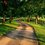# [Number Theory] Proving special cases of Dirichlet's Theorem

Let's prove that there are infinitely many primes $p$ such that $p \equiv 3~ (mod~4)$.

Let $p_1, p_2, ... p_k$ be a finite list of primes congruent to 3 mod 4, and construct a new integer

$4 \times (p_1, p_2, ... p_k) -1$

that is 3 mod 4, and not divisible by any of the $p_i$'s, $( 0.

Note that $4 \times (p_1, p_2, ... p_k) -1$ is an odd integer, so it can only have prime factors that are 1 or 3 mod 4. Let's suppose that all its prime factors were 1 and 4. Then

$1\cdot 1 \cdot 1 \cdots 1=1 ~mod~ 4,$

so $4 \times (p_1, p_2, ... p_k) -1$ would also be 1 mod 4, but this is a contradiction, because our integer is 3 mod 4 by construction. So there must be at least one prime factor that is 3 mod 4. But since $4 \times (p_1, p_2, ... p_k) -1$ is not divisible by any $p_i$, which are primes congruent to 3 mod 4, we have thus found a new prime $p_{k+1} = 3~mod~ 4$ that is not in our original list $p_1, p_2, ... p_k$.

$\therefore$ there must be an infinitude of primes that are congruent to $3 ~mod ~4$.

Another special case is that there exists infinitely many primes $p$ such that $p \equiv 1~(mod~4)$. This case can be shown to be true in the same way, by constructing an integer $(p_1p_2 \cdots p_t)^2 +1$, and following the same procedure above.

In fact, Dirichlet's theorem states that for $(k,m)=1$, any coprime integers $m$ and $k$, there exists infinitely many primes $p$ such that $p \equiv m ~(mod ~k)$. His own proof, consisting of Dirichlet L-functions, is often considered to have contributed to the origins of Analytic Number Theory.Note by Bright Glow
3 years, 2 months ago

This discussion board is a place to discuss our Daily Challenges and the math and science related to those challenges. Explanations are more than just a solution — they should explain the steps and thinking strategies that you used to obtain the solution. Comments should further the discussion of math and science.

When posting on Brilliant:

• Use the emojis to react to an explanation, whether you're congratulating a job well done , or just really confused .
• Ask specific questions about the challenge or the steps in somebody's explanation. Well-posed questions can add a lot to the discussion, but posting "I don't understand!" doesn't help anyone.
• Try to contribute something new to the discussion, whether it is an extension, generalization or other idea related to the challenge.

MarkdownAppears as
*italics* or _italics_ italics
**bold** or __bold__ bold
- bulleted- list
• bulleted
• list
1. numbered2. list
1. numbered
2. list
Note: you must add a full line of space before and after lists for them to show up correctly
paragraph 1paragraph 2

paragraph 1

paragraph 2

[example link](https://brilliant.org)example link
> This is a quote
This is a quote
    # I indented these lines
# 4 spaces, and now they show
# up as a code block.

print "hello world"
# I indented these lines
# 4 spaces, and now they show
# up as a code block.

print "hello world"
MathAppears as
Remember to wrap math in $$ ... $$ or $ ... $ to ensure proper formatting.
2 \times 3 $2 \times 3$
2^{34} $2^{34}$
a_{i-1} $a_{i-1}$
\frac{2}{3} $\frac{2}{3}$
\sqrt{2} $\sqrt{2}$
\sum_{i=1}^3 $\sum_{i=1}^3$
\sin \theta $\sin \theta$
\boxed{123} $\boxed{123}$